# How to generate random values from a distribution

## Description

There are many famous continuous probability distributions, such as the normal and exponential distributions. How can we get access to them in software, to generate random values from a chosen distribution?

## Solution, in Excel

View this solution alone.

You can generate random numbers from many common distributions easily using the Data Analysis Toolpak. (Below we cover another method that does not use the Data Analysis Toolpak.) If you’ve never enabled it before, see these instructions from Microsoft on how to do so.

On the Data tab, click the Data Analysis button, shown below.From the list of tools it provides, choose Random Number Generation, as shown below, then click OK.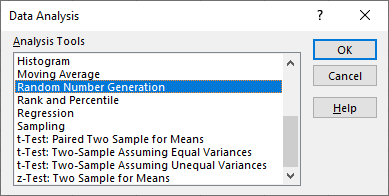Choose a number of variables (that is, columns of output) and of random numbers (that is, rows of output) and a distribution. Once you select a distribution, you can also select its parameters (e.g., the mean and standard deviation for a normal distribution). Choose where you want the output and then click OK.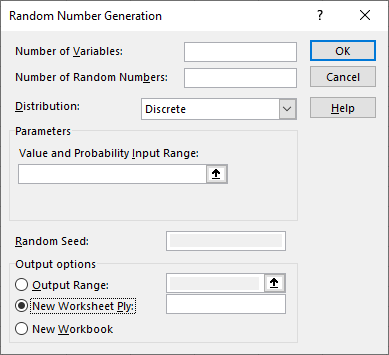Here is example data generated for 3 variables, 20 random numbers per variable, using a standard normal distribution.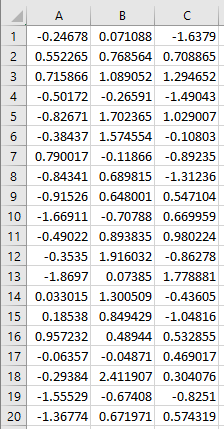It’s also possible to generate random numbers using Excel formulas in place of the Data Analysis Toolpak. Here’s how:

No matter what distribution you want to draw from, begin by generating random values from the uniform distribution on the interval [0,1] using the =RAND() function. For example, if you’ll want 10 random values, place the =RAND() formula into 10 cells in a single column, like so: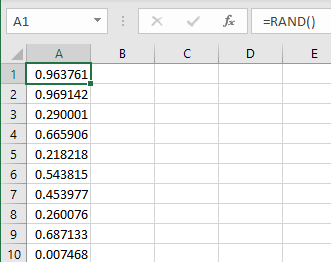Then in an adjacent column, apply one of the built-in inverse CDF functions from Excel’s statistics function set. For example, to generate values from a normal distribution with mean 5 and standard deviation 2, apply =NORM.INV(_,5,2) to each random number in the first column. The NORM.INV function converts uniform random values into random values chosen from the specified distribution.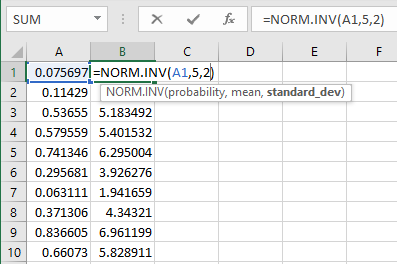Excel also has built-in functions for several other distributions, including BETA.INV, BINOM.INV, CHISQ.INV, F.INV, GAMMA.INV, LOGNORM.INV, and T.INV.

Excel recomputes random values every time a formula or cell changes. If you do not want this behavior, simply copy all the random cells and then paste them back into the exact same locations, but using the “Paste Values” functionality of Excel, which removes the original formulas, leaving only their final results.

See a problem? Tell us or edit the source.

## Solution, in Julia

View this solution alone.

You can import many different random variables from Julia’s Distributions package. The full list of them is online here.

If you don’t have that package installed, first run using Pkg and then Pkg.add( "Distributions" ) from within Julia.

Regardless of whether the distribution is discrete or continuous, the appropriate function to call is rand. Here are two examples.

Using a normal distribution:

1
2
3
using Distributions
X = Normal( 5, 3 )
rand( X, 10 )

1
2
3
4
5
6
7
8
9
10
11
10-element Vector{Float64}:
2.18036354985213
4.261755639220276
9.175724974437623
7.111178500969482
5.784059237346303
2.276916458848387
4.323059921916803
7.067942300207913
5.040815993440384
5.401080085074974


Using a uniform distribution:

In this example, we generate the random values in one line of code, without giving the random variable a name.

1
2
using Distributions
rand( Uniform( 100, 200 ), 5 )

1
2
3
4
5
6
5-element Vector{Float64}:
120.34366617283129
117.18012200542422
121.03058480958376
140.31797801233535
109.153400454394


See a problem? Tell us or edit the source.

## Using SciPy, in Python

View this solution alone.

You can import many different random variables from SciPy’s stats module. The full list of them is online here.

Regardless of whether the distribution is discrete or continuous, the appropriate function to call is rvs, which stands for “random values.” Here are two examples.

Using a normal distribution:

1
2
3
from scipy import stats
X = stats.norm( 10, 5 )  # normal random variable with μ=10 and σ=5
X.rvs( 20 )              # 20 random values from X

1
2
3
4
array([10.6907129 , 14.18269263, 11.81631776,  8.01109692, 13.02531043,
7.81131811, 13.28578636, 11.24026458, 11.15153426, 17.88676989,
19.31140617,  9.6059965 , 12.1120152 , 19.4371871 , 11.20087368,
8.82303356, 20.84662811,  0.3140319 , 16.45965892,  8.64633779])


Using a uniform distribution:

(Note that in SciPy, the uniform distribution needs a “location,” which is where the sample space begins—in this case 50—and a “scale,” which is the width of the sample space—in this case 10.)

1
2
3
from scipy import stats
X = stats.uniform( 50, 10 )  # uniform random variable on the interval [50,60]
X.rvs( 20 )                  # 20 random values from X

1
2
3
4
array([55.45216751, 51.33233834, 52.95952577, 50.73167814, 58.03758018,
51.92018223, 56.50131882, 51.17126188, 54.57665328, 57.67945112,
52.70825309, 56.02047417, 59.47625062, 52.09755942, 54.7246222 ,
54.71473066, 59.81365965, 59.2618776 , 54.9747678 , 50.74177568])


See a problem? Tell us or edit the source.

## Solution, in R

View this solution alone.

Because R is designed for use in statistics, it comes with many probability distributions built in. A list of them is online here.

Regardless of whether the distribution is discrete or continuous, prefix the name of the distribution with r, which stands for “random values.” Here are two examples.

Using a normal distribution:

1
2
# 20 random values from the normal distribution with μ=10 and σ=5
rnorm( 20, mean=10, sd=5 )

1
2
3
  8.281648  9.853892 16.533054 15.195100 10.301387  8.169758 -2.927952
  9.463419  6.168776 15.666091 13.382661  4.286710 11.340385  6.448717
  9.148462 11.744665  8.869667 13.177116  6.309141  8.888176


Using a uniform distribution:

1
2
# 20 random values from the uniform distribution on the interval [50,60]
runif( 20, min=50, max=60 )

1
2
3
 59.59391 59.85593 54.76225 57.33802 54.03049 52.52659 51.66029 58.05590
 56.11249 53.00606 58.47839 52.03311 54.31438 57.61727 53.04272 55.41182
 51.47592 59.49853 55.94943 58.30232